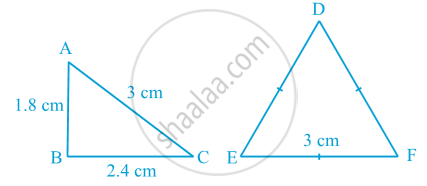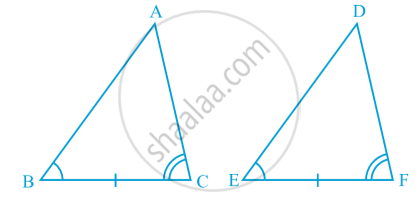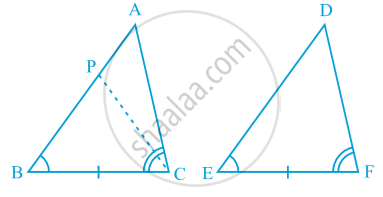Share

# Criteria for Congruence of Triangles

Course

#### text

For instance, Draw two triangles with one side 3 cm. Are these triangles are not  congruent in following fig .Axiom (SAS congruence rule) : Two triangles are congruent if two sides and the included angle of one triangle are equal to the two sides and the included angle of the other triangle.

#### theorem

Theorem (ASA congruence rule) :  Two triangles are congruent if two angles and the included side of one triangle are equal to two angles and the included side of other triangle.
Proof : We are given two triangles ABC and DEF in which:
∠ B = ∠ E, ∠ C = ∠ F
and BC = EF
We need to prove that   ∆ ABC ≅ ∆ DEF
For proving the congruence of the two triangles see that three cases arise.

Case (i) : Let AB = DE in following fig.You may observe that
AB = DE (Assumed)
∠ B = ∠ E (Given)
BC = EF (Given)
So, ∆ ABC ≅ ∆ DEF   (By SAS rule)

Case (ii) :
Let if possible AB > DE. So, we can take a point P on AB such that PB = DE. Now consider ∆ PBC and ∆ DEF  in following fig.Observe that in ∆ PBC and ∆ DEF,
PB = DE (By construction)
∠ B = ∠ E (Given)
BC = EF (Given)
So, we can conclude that:
∆ PBC ≅ ∆ DEF, by the SAS axiom for congruence.
Since the triangles are congruent, their corresponding parts will be equal.
So, ∠ PCB = ∠ DFE
But, we are given that
∠ ACB = ∠ DFE So, ∠ ACB = ∠ PCB
This is possible only if P coincides with A.
or, BA = ED
So, ∆ ABC ≅ ∆ DEF   (by SAS axiom)

Case (iii) :  If AB < DE, we can choose a point M on DE such that ME = AB and repeating the arguments as given in Case (ii),  we can conclude that AB = DE and so, ∆ ABC ≅ ∆ DEF.
You know that the sum of the three angles of a triangle is 180°. So if two pairs of angles are equal, the third pair is also equal (180° – sum of equal angles).
So, two triangles are congruent if any two pairs of angles and one pair of corresponding sides are equal. We may call it as the AAS Congruence Rule.

### Shaalaa.com

Axiom : SAS (Side-Angle-Side) [00:14:07]
S
0%

S